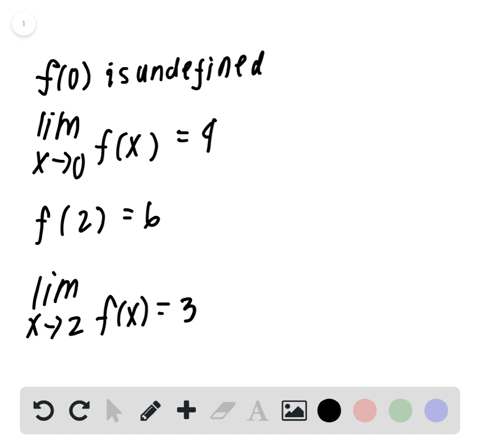Enroll in one of our FREE online STEM summer camps. Space is limited so join now!View Summer Courses### Sketching a Graph In Exercises 33 and $34,$ sketc…

01:32University of California, Berkeley

Need more help? Fill out this quick form to get professional live tutoring.

Get live tutoring
Problem 32

In Exercises 31 and $32,$ sketch the graph of $f$ . Then identify the values of $c$ for which $$\lim _{x \rightarrow e} f(x)$$

$$f(x)=\left\{\begin{array}{ll}{\sin x,} & {x < 0} \\ {1-\cos x,} & {0 \leq x<\pi} \\ {\cos x,} & {x>\pi}\end{array}\right.$$

## Discussion

You must be signed in to discuss.

## Video Transcript

Okay, so we have to find the limits. Exact approaches. E of car function. I said this value should be you. Yeah, I believe it. He not be. And we have our people function in here. So this is Ah, piece. I function. So when x is less than zero, we have sign of X when X is between zero and pi live with my school center. But And when co sign for one extra created entirely, of course. In fact, where to identified the values of sea for which the limits in this. Okay, so we can see that the function here as a break right here, which is this is that pie so basically limited this for all valleys of sea except her at pipe at this point where when we approached pie from the left, the limit approaches to So this was too right here. And then when we're putting from the right, we approached a negative one. It's re approach different values from the left and right. The limit does not exist when C is equal to fly. So we didn't see no money could buy, but it's limited